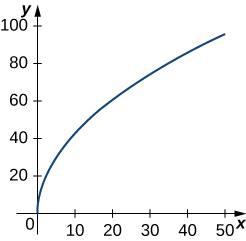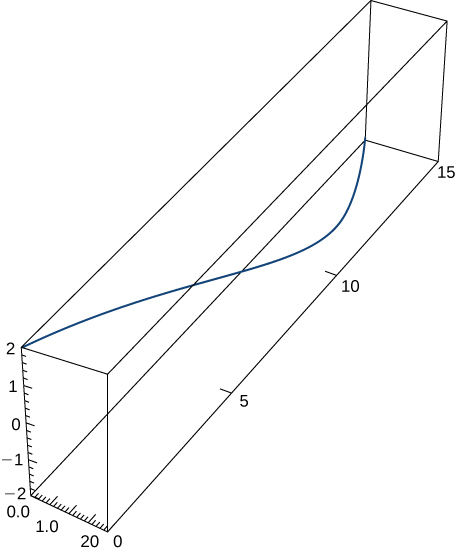$$\newcommand{\id}{\mathrm{id}}$$ $$\newcommand{\Span}{\mathrm{span}}$$ $$\newcommand{\kernel}{\mathrm{null}\,}$$ $$\newcommand{\range}{\mathrm{range}\,}$$ $$\newcommand{\RealPart}{\mathrm{Re}}$$ $$\newcommand{\ImaginaryPart}{\mathrm{Im}}$$ $$\newcommand{\Argument}{\mathrm{Arg}}$$ $$\newcommand{\norm}{\| #1 \|}$$ $$\newcommand{\inner}{\langle #1, #2 \rangle}$$ $$\newcommand{\Span}{\mathrm{span}}$$

# 12.4E: Exercises for Section 12.4

$$\newcommand{\vecs}{\overset { \rightharpoonup} {\mathbf{#1}} }$$ $$\newcommand{\vecd}{\overset{-\!-\!\rightharpoonup}{\vphantom{a}\smash {#1}}}$$$$\newcommand{\id}{\mathrm{id}}$$ $$\newcommand{\Span}{\mathrm{span}}$$ $$\newcommand{\kernel}{\mathrm{null}\,}$$ $$\newcommand{\range}{\mathrm{range}\,}$$ $$\newcommand{\RealPart}{\mathrm{Re}}$$ $$\newcommand{\ImaginaryPart}{\mathrm{Im}}$$ $$\newcommand{\Argument}{\mathrm{Arg}}$$ $$\newcommand{\norm}{\| #1 \|}$$ $$\newcommand{\inner}{\langle #1, #2 \rangle}$$ $$\newcommand{\Span}{\mathrm{span}}$$ $$\newcommand{\id}{\mathrm{id}}$$ $$\newcommand{\Span}{\mathrm{span}}$$ $$\newcommand{\kernel}{\mathrm{null}\,}$$ $$\newcommand{\range}{\mathrm{range}\,}$$ $$\newcommand{\RealPart}{\mathrm{Re}}$$ $$\newcommand{\ImaginaryPart}{\mathrm{Im}}$$ $$\newcommand{\Argument}{\mathrm{Arg}}$$ $$\newcommand{\norm}{\| #1 \|}$$ $$\newcommand{\inner}{\langle #1, #2 \rangle}$$ $$\newcommand{\Span}{\mathrm{span}}$$

## Determining Arc Length

In questions 1 - 5, find the arc length of the curve on the given interval.

1) $$\vecs r(t)=t^2 \,\hat{\mathbf{i}}+(2t^2+1)\,\hat{\mathbf{j}}, \quad 1≤t≤3$$

$$8\sqrt{5}$$ units

2) $$\vecs r(t)=t^2 \,\hat{\mathbf{i}}+14t \,\hat{\mathbf{j}},\quad 0≤t≤7$$. This portion of the graph is shown here:3) $$\vecs r(t)=⟨t^2+1,4t^3+3⟩, \quad −1≤t≤0$$

$$\frac{1}{54}(37^{3/2}−1)$$ units

4) $$\vecs r(t)=⟨2 \sin t,5t,2 \cos t⟩,\quad 0≤t≤π$$. This portion of the graph is shown here:5) $$\vecs r(t)=⟨e^{−t \cos t},e^{−t \sin t}⟩$$ over the interval $$[0,\frac{π}{2}]$$. Here is the portion of the graph on the indicated interval:6) Set up an integral to represent the arc length from $$t = 0$$ to $$t = 2$$ along the curve traced out by $$\vecs r(t) = \langle t, \, t^4\rangle.$$ Then use technology to approximate this length to the nearest thousandth of a unit.

7) Find the length of one turn of the helix given by $$\vecs r(t)= \frac{1}{2} \cos t \,\hat{\mathbf{i}}+\frac{1}{2} \sin t \,\hat{\mathbf{j}}+\frac{\sqrt{3}}{2}\,t \,\hat{\mathbf{k}}$$.

Length $$=2π$$ units

8) Find the arc length of the vector-valued function $$\vecs r(t)=−t \,\hat{\mathbf{i}}+4t \,\hat{\mathbf{j}}+3t \,\hat{\mathbf{k}}$$ over $$[0,1]$$.

9) A particle travels in a circle with the equation of motion $$\vecs r(t)=3 \cos t \,\hat{\mathbf{i}}+3 \sin t \,\hat{\mathbf{j}} +0 \,\hat{\mathbf{k}}$$. Find the distance traveled around the circle by the particle.

$$6π$$ units

10) Set up an integral to find the circumference of the ellipse with the equation $$\vecs r(t)= \cos t \,\hat{\mathbf{i}}+2 \sin t \,\hat{\mathbf{j}}+0\,\hat{\mathbf{k}}$$.

11) Find the length of the curve $$\vecs r(t)=⟨\sqrt{2}t,\, e^t, \, e^{−t}⟩$$ over the interval $$0≤t≤1$$. The graph is shown here:$$\left(e−\frac{1}{e}\right)$$ units

12) Find the length of the curve $$\vecs r(t)=⟨2 \sin t, \, 5t, \, 2 \cos t⟩$$ for $$t∈[−10,10]$$.

## Unit Tangent Vectors and Unit Normal Vectors

13) The position function for a particle is $$\vecs r(t)=a \cos( ωt) \,\hat{\mathbf{i}}+b \sin (ωt) \,\hat{\mathbf{j}}$$. Find the unit tangent vector and the unit normal vector at $$t=0$$.

Solution:
\begin{align*} \vecs r'(t) &= -aω \sin( ωt) \,\hat{\mathbf{i}}+bω \cos (ωt) \,\hat{\mathbf{j}}\\[5pt] \| \vecs r'(t) \| &= \sqrt{a^2 ω^2 \sin^2(ωt) +b^2ω^2\cos^2(ωt)} \\[5pt] \vecs T(t) &= \dfrac{\vecs r'(t)}{\| \vecs r'(t) \| } = \dfrac{-aω \sin( ωt) \,\hat{\mathbf{i}}+bω \cos (ωt) \,\hat{\mathbf{j}}}{\sqrt{a^2 ω^2 \sin^2(ωt) +b^2ω^2\cos^2(ωt)}}\\[5pt] \vecs T(0) &= \dfrac{bω \,\hat{\mathbf{j}}}{\sqrt{(bω)^2}} = \dfrac{bω \,\hat{\mathbf{j}}}{|bω|}\end{align*}

If $$bω > 0, \; \vecs T(0) = \hat{\mathbf{j}},$$   and if   $$bω < 0, \; T(0)= -\hat{\mathbf{j}}$$
If $$bω > 0, \; \vecs T(0)= \hat{\mathbf{j}},$$   and if   $$bω < 0, \; \vecs T(0)= -\hat{\mathbf{j}}$$

If $$a > 0, \; \vecs N(0)= -\hat{\mathbf{i}},$$   and if   $$a < 0, \; \vecs N(0)= \hat{\mathbf{i}}$$

14) Given $$\vecs r(t)=a \cos (ωt) \,\hat{\mathbf{i}} +b \sin (ωt) \,\hat{\mathbf{j}}$$, find the binormal vector $$\vecs B(0)$$.

15) Given $$\vecs r(t)=⟨2e^t,e^t \cos t,e^t \sin t⟩$$, determine the unit tangent vector $$\vecs T(t)$$.

\begin{align*} \vecs T(t) &=\left\langle \frac{2}{\sqrt{6}},\, \frac{\cos t− \sin t}{\sqrt{6}}, \, \frac{\cos t+ \sin t}{\sqrt{6}}\right\rangle \\[4pt] &= \left\langle\frac{\sqrt{6}}{3},\, \frac{\sqrt{6}}{6} (\cos t− \sin t), \, \frac{\sqrt{6}}{6} (\cos t+ \sin t)\right\rangle \end{align*}

16) Given $$\vecs r(t)=⟨2e^t,\, e^t \cos t,\, e^t \sin t⟩$$, find the unit tangent vector $$\vecs T(t)$$ evaluated at $$t=0$$, $$\vecs T(0)$$.

17) Given $$\vecs r(t)=⟨2e^t,\, e^t \cos t,\, e^t \sin t⟩$$, determine the unit normal vector $$\vecs N(t)$$.

$$\vecs N(t)=⟨0,\, -\frac{\sqrt{2}}{2} (\sin t + \cos t), \, \frac{\sqrt{2}}{2} (\cos t- \sin t)⟩$$

18) Given $$\vecs r(t)=⟨2e^t,\, e^t \cos t,\, e^t \sin t⟩$$, find the unit normal vector $$\vecs N(t)$$ evaluated at $$t=0$$, $$\vecs N(0)$$.

$$\vecs N(0)=⟨0, \;-\frac{\sqrt{2}}{2},\;\frac{\sqrt{2}}{2}⟩$$

19) Given $$\vecs r(t)=t \,\hat{\mathbf{i}}+t^2 \,\hat{\mathbf{j}}+t \,\hat{\mathbf{k}}$$, find the unit tangent vector $$\vecs T(t)$$. The graph is shown here:$$\vecs T(t)=\dfrac{1}{\sqrt{4t^2+2}}<1,2t,1>$$

20) Find the unit tangent vector $$\vecs T(t)$$ and unit normal vector $$\vecs N(t)$$ at $$t=0$$ for the plane curve $$\vecs r(t)=⟨t^3−4t,5t^2−2⟩$$. The graph is shown here:21) Find the unit tangent vector $$\vecs T(t)$$ for $$\vecs r(t)=3t \,\hat{\mathbf{i}}+5t^2 \,\hat{\mathbf{j}}+2t \,\hat{\mathbf{k}}$$.

$$\vecs T(t)=\dfrac{1}{\sqrt{100t^2+13}}(3 \,\hat{\mathbf{i}}+10t \,\hat{\mathbf{j}}+2 \,\hat{\mathbf{k}})$$

22) Find the principal normal vector to the curve $$\vecs r(t)=⟨6 \cos t,6 \sin t⟩$$ at the point determined by $$t=\frac{π}{3}$$.

23) Find $$\vecs T(t)$$ for the curve $$\vecs r(t)=(t^3−4t) \,\hat{\mathbf{i}}+(5t^2−2) \,\hat{\mathbf{j}}$$.

$$\vecs T(t)=\dfrac{1}{\sqrt{9t^4+76t^2+16}}\big((3t^2−4)\,\hat{\mathbf{i}}+10t \,\hat{\mathbf{j}}\big)$$

24) Find $$\vecs N(t)$$ for the curve $$\vecs r(t)=(t^3−4t)\,\hat{\mathbf{i}}+(5t^2−2)\,\hat{\mathbf{j}}$$.

25) Find the unit tangent vector $$\vecs T(t)$$ for $$\vecs r(t)=⟨2 \sin t,\, 5t,\, 2 \cos t⟩$$.

$$\vecs T(t)=⟨\frac{2\sqrt{29}}{29}\cos t,\, \frac{5\sqrt{29}}{29},\,−\frac{2\sqrt{29}}{29}\sin t⟩$$

26) Find the unit normal vector $$\vecs N(t)$$ for $$\vecs r(t)=⟨2\sin t,\,5t,\,2\cos t⟩$$.

$$\vecs N(t)=⟨−\sin t,\, 0,\, −\cos t⟩$$

## Arc Length Parameterizations

27) Find the arc-length function $$\vecs s(t)$$ for the line segment given by $$\vecs r(t)=⟨3−3t,\, 4t⟩$$. Then write the arc-length parameterization of $$r$$ with $$s$$ as the parameter.

Arc-length function: $$s(t)=5t$$; The arc-length parameterization of $$\vecs r(t)$$: $$\vecs r(s)=\left(3−\dfrac{3s}{5}\right)\,\hat{\mathbf{i}}+\dfrac{4s}{5}\,\hat{\mathbf{j}}$$

28) Parameterize the helix $$\vecs r(t)= \cos t \,\hat{\mathbf{i}}+ \sin t \,\hat{\mathbf{j}}+t \,\hat{\mathbf{k}}$$ using the arc-length parameter $$s$$, from $$t=0$$.

29) Parameterize the curve using the arc-length parameter $$s$$, at the point at which $$t=0$$ for $$\vecs r(t)=e^t \sin t \,\hat{\mathbf{i}} + e^t \cos t \,\hat{\mathbf{j}}$$

$$\vecs r(s)=\left(1+\dfrac{s}{\sqrt{2}}\right) \sin \left( \ln \left(1+ \dfrac{s}{\sqrt{2}}\right)\right)\,\hat{\mathbf{i}} +\left(1+ \dfrac{s}{\sqrt{2}}\right) \cos \left( \ln \left(1+\dfrac{s}{\sqrt{2}}\right)\right)\,\hat{\mathbf{j}}$$

## Curvature and the Osculating Circle

30) Find the curvature of the curve $$\vecs r(t)=5 \cos t \,\hat{\mathbf{i}}+4 \sin t \,\hat{\mathbf{j}}$$ at $$t=π/3$$. (Note: The graph is an ellipse.)31) Find the $$x$$-coordinate at which the curvature of the curve $$y=1/x$$ is a maximum value.

The maximum value of the curvature occurs at $$x=1$$.

32) Find the curvature of the curve $$\vecs r(t)=5 \cos t \,\hat{\mathbf{i}}+5 \sin t \,\hat{\mathbf{j}}$$. Does the curvature depend upon the parameter $$t$$?

33) Find the curvature $$κ$$ for the curve $$y=x−\frac{1}{4}x^2$$ at the point $$x=2$$.

$$\frac{1}{2}$$

34) Find the curvature $$κ$$ for the curve $$y=\frac{1}{3}x^3$$ at the point $$x=1$$.

35) Find the curvature $$κ$$ of the curve $$\vecs r(t)=t \,\hat{\mathbf{i}}+6t^2 \,\hat{\mathbf{j}}+4t \,\hat{\mathbf{k}}$$. The graph is shown here:$$κ≈\dfrac{49.477}{(17+144t^2)^{3/2}}$$

36) Find the curvature of $$\vecs r(t)=⟨2 \sin t,5t,2 \cos t⟩$$.

37) Find the curvature of $$\vecs r(t)=\sqrt{2}t \,\hat{\mathbf{i}}+e^t \,\hat{\mathbf{j}}+e^{−t} \,\hat{\mathbf{k}}$$ at point $$P(0,1,1)$$.

$$\frac{1}{2\sqrt{2}}$$

38) At what point does the curve $$y=e^x$$ have maximum curvature?

39) What happens to the curvature as $$x→∞$$ for the curve $$y=e^x$$?

The curvature approaches zero.

40) Find the point of maximum curvature on the curve $$y=\ln x$$.

41) Find the equations of the normal plane and the osculating plane of the curve $$\vecs r(t)=⟨2 \sin (3t),t,2 \cos (3t)⟩$$ at point $$(0,π,−2)$$.

$$y=6x+π$$ and $$x+6y=6π$$

42) Find equations of the osculating circles of the ellipse $$4y^2+9x^2=36$$ at the points $$(2,0)$$ and $$(0,3)$$.

43) Find the equation for the osculating plane at point $$t=π/4$$ on the curve $$\vecs r(t)=\cos (2t) \,\hat{\mathbf{i}}+ \sin (2t) \,\hat{\mathbf{j}}+t\,\hat{\mathbf{k}}$$.

$$x+2z=\frac{π}{2}$$

44) Find the radius of curvature of $$6y=x^3$$ at the point $$(2,\frac{4}{3}).$$

45) Find the curvature at each point $$(x,y)$$ on the hyperbola $$\vecs r(t)=⟨a \cosh( t),b \sinh (t)⟩$$.

$$\dfrac{a^4b^4}{(b^4x^2+a^4y^2)^{3/2}}$$

46) Calculate the curvature of the circular helix $$\vecs r(t)=r \sin (t) \,\hat{\mathbf{i}}+r \cos (t) \,\hat{\mathbf{j}}+t \,\hat{\mathbf{k}}$$.

47) Find the radius of curvature of $$y= \ln (x+1)$$ at point $$(2,\ln 3)$$.

$$\frac{10\sqrt{10}}{3}$$

48) Find the radius of curvature of the hyperbola $$xy=1$$ at point $$(1,1)$$.

A particle moves along the plane curve $$C$$ described by $$\vecs r(t)=t \,\hat{\mathbf{i}}+t^2 \,\hat{\mathbf{j}}$$. Use this parameterization to answer questions 49 - 51.

49) Find the length of the curve over the interval $$[0,2]$$.

$$\frac{1}{4}\big[ 4\sqrt{17} + \ln\left(4+\sqrt{17}\right)\big]\text{ units }\approx 4.64678 \text{ units}$$

50) Find the curvature of the plane curve at $$t=0,1,2$$.

51) Describe the curvature as t increases from $$t=0$$ to $$t=2$$.

The curvature is decreasing over this interval.

The surface of a large cup is formed by revolving the graph of the function $$y=0.25x^{1.6}$$ from $$x=0$$ to $$x=5$$ about the $$y$$-axis (measured in centimeters).

52) [T] Use technology to graph the surface.

53) Find the curvature $$κ$$ of the generating curve as a function of $$x$$.

$$κ=\dfrac{30}{x^{2/5}\left(25+4x^{6/5}\right)^{3/2}}$$

$$\dfrac{6}{25x^{2/5}\left(1+\frac{4}{25}x^{6/5}\right)^{3/2}}$$

We can simplify it as follows:
\begin{align*} \dfrac{6}{25x^{2/5}\left(1+\frac{4}{25}x^{6/5}\right)^{3/2}} &= \dfrac{6}{25x^{2/5}\big[\frac{1}{25}\left(25+4x^{6/5}\right)\big]^{3/2}}\\[4pt] &= \dfrac{6}{25x^{2/5}\left(\frac{1}{25}\right)^{3/2}\big[25+4x^{6/5}\big]^{3/2}} \\[4pt] &= \dfrac{6}{\frac{25}{125}x^{2/5}\big[25+4x^{6/5}\big]^{3/2}} \\[4pt] &= \dfrac{30}{x^{2/5}\left(25+4x^{6/5}\right)^{3/2}}\end{align*}

54) [T] Use technology to graph the curvature function.